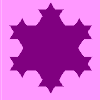#### You may also like### Is There a Theorem?

Draw a square. A second square of the same size slides around the first always maintaining contact and keeping the same orientation. How far does the dot travel?### Coins on a Plate

Points A, B and C are the centres of three circles, each one of which touches the other two. Prove that the perimeter of the triangle ABC is equal to the diameter of the largest circle.### Smaller and Smaller

Can you predict, without drawing, what the perimeter of the next shape in this pattern will be if we continue drawing them in the same way?

# On the Edge

### Why dothis problem?

This problem is a familar context involving perimeters and squares that requires careful analysis. There are opportunities to experiment but a need to think ahead.

### Possible approach

Arrange four large squares into a $2 \times 2$ array. Colour the edges - counting from $1$ to $8$ as you go along.
Rearrange the four squares to enable you to colour the edges in a different colour (without repeat and counting $1$ to $8$ again).

Arrange nine large squares into a $3 \times 3$ array and colour the edges - counting from $1$ to $12$ this time.

Rearrange the nine squares in a haphazard way to enable you to colour the edges in a different colour (counting $1$ to $12$).

"I wonder whether we might be able to colour the edge in a third colour?"

Rearrange the nine squares again and "discover" that you are not going to be able to do it - at least not on this occasion.

"Does this mean we cannot do it?"

or

"What went wrong?"
or
"Does this mean it is impossible?"

Look out for responses that develop the idea that you need to be able to draw $12$ lines and there are $12$ "blank" edges. However, not all of these are on the edge of the new array.

"So it feels like it should be possible with a bit more thought".

Hand out sets of paper squares to pairs of students and challenge them to complete the task successfully.

When any pair is successful challenge them to repeat the task:

"Well done. Would you be able to do this again without making any "false moves"?"

After the first colouring, can students position the squares for the second colouring knowing it will work for the third?

Move on to larger square arrays.

"Can we use more colours when we have $16$ squares?"

"How about with $25$ squares...?"

### Key questions

Can you anticipate where certain squares will have to go in future rounds?

### Possible support

Lots of paper squares so students do not have to worry about making mistakes.

A $4 \times 4$ array is probably easier.

### Possible extension

Can students do all three colourings without rearranging the squares?

Will it always be possible to add an extra colour as the squares get larger?

For a 3D version of this problem students could try Inside Out